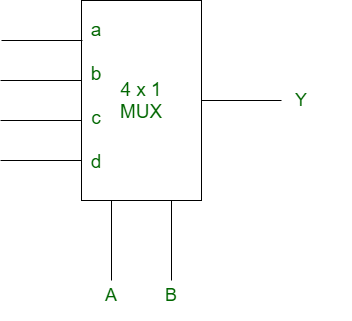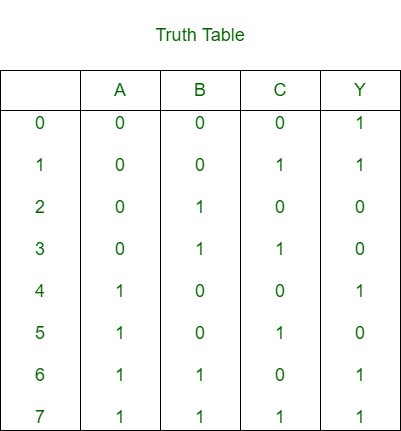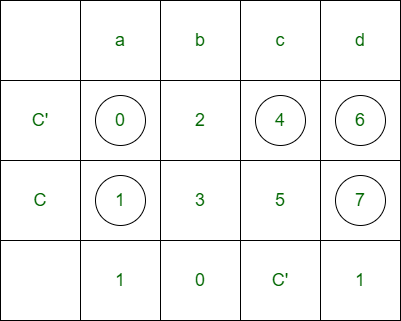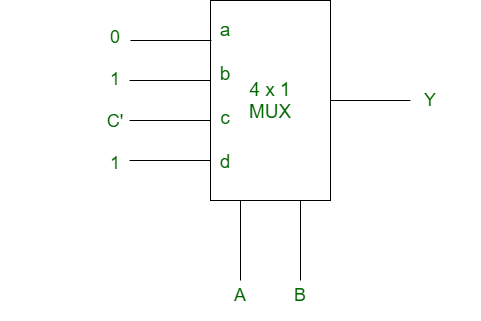# Implementation of SOP function using multiplexer

Given a SOP function and a multiplexer is also given. We will need to implement the given SOP function using the given MUX. There are certain steps involved in it: Step 1: Draw the truth table for the given number of variable function. Step 2: Consider one variable as input and remaining variables as select lines. Step 3: Form a matrix where input lines of MUX are columns and input variable and its complement are rows. Step 4: Find AND between both rows on the basis of the truth table. Step 5: Hence whatever is found is considered as input of MUX. We will illustrate it with an example: Example: Given SOP function f(A, B, C) = m(0, 1, 4, 6, 7) and MUX isFor 3 variable function, the truth table isLet A and B are the select lines and C be the input,Thus, for the implementation of given logical function, required is one 4×1 MUX and and inverter.